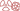THE PHENOMENON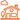IN DAILY LIFE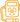QUESTION OF DOSES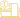AT THE DOCTOR’S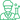At the laboratory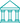At the museum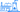NUCLEAR ENERGY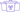A general public knowledge base dedicated to radioactivity created and maintained by the french physics community

# Gamma Attenuation

## Attenuation of a gamma beam

Of the three types of radiations, gamma rays are the most penetrative and therefore the most difficult to avoid. In theory, they can never be completely stopped, but only attenuated. In practice, however, severe attenuation is as good as a cut-off. This objective is achieved by most of the protective screens in radioprotection..

The attenuation with a screen comes in addition to the solid angle effect, which is purely geometrical and states that the number of photons hitting a target in vacuum drops as the square of the distance to the source.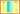Gamma interaction and attenuation:
A gamma beam attenuation is defined as the fraction of gammas which come through an absorber without interacting. Of the 6 gammas represented, only A and C emerge unaffected. B, E and F are totally absorbed by the screen, whereas gamma ray D is partially absorbed. Gamma D is not counted as it emerges from the absorber as a Compton photon, travelling in a different direction with a lower energy. These Compton photons should be counted, however, when ‘absorption’ of gamma rays are considered.

When a gamma photon passes through a portion of screen, it does not deposit gradually its energy as an alpha particle on its way : either it interacts and disappears as such, or passes all the way through without interacting. In the case of a large number of gamma – a beam – a number of gamma emerge unscathed. The beam is attenuated.

The stronger the ‘probability of interaction’, the faster the attenuation. The attenuation coefficient of an absorber is in proportion of this interaction probability, called ‘cross-section’. This attenuation coefficient plays the role of stopping power and replaces the energy loss by ionization that slows down alpha or beta particles.

In the simple case where mono-energetic gamma rays interact with a homogenous screen, the attenuation would be constant at any given depth in the absorber . The intensity of radiation decreases exponentially with the thickness of the screen – similar to the way in which the activity of a radioactive sample drops over time. This similarity allows us to define an ‘attenuation length’ (the inverse of the attenuation coefficient) : the ‘attenuation length’ is mathematically similar to the half-life in radioactive decay (the intensity halves after every such distance).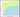Gamma attenuation lengths in water and lead
For gamma radiation, the attenuation length is the inverse of the attenuation coefficient. The shorter the length, the faster the attenuation. Less than a millimetre of lead is enough to halve the number of gamma rays with an energy below 200 keV, whereas it would take over 5cm of water to achieve the same effect. Lead is less effective, however, when it comes to gamma rays with energies over 1 MeV: 1cm of lead is needed as opposed to 10 cm of water.  lead is 11.6 times denser than water).

This characteristic attenuation length varies greatly with the nature of the absorber and the energy of the gamma rays involved. The ‘attenuation length’ and depths are often expressed in grams/cm2 instead of cm, which allows to compare more easily absorbers made of different substances.

Comparing the attenuation capacity of water and lead at equal mass (with densities of 1.0 and 11.6), water is much less efficient for soft gamma rays but its performance improves for at higher energies close to 1 MeV. Lead is particularly effective for attenuating X-rays and other photons of low energies, as its attenuation length is under a millimetre. For gamma rays at the high end of the energy spectrum, however, it would take nearly a centimetre of lead to halve the number of rays.

NEXT : Gamma AbsorptionTHE PHENOMENONIN DAILY LIFEQUESTION OF DOSESAT THE DOCTOR’SAt the laboratoryAt the museumNUCLEAR ENERGY